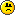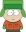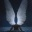Coffeehouse for desis
Would you like to react to this message? Create an account in a few clicks or log in to continue.

# Middle School Math Quiz

3 posters##Middle School Math Quiz

Relatively easy Middle School quiz problems from a math magazine. Please explain your answers (no need for a big narrative, just a short explanation will do).

1. John picked a chrysanthemum with a Fibonacci number of petals. He plucked one petal: “She loves me.” He plucked a second petal: “She loves me not.” He plucked a third petal: “She loves me.” He continued in this way until he reached the last petal. What is the probability that the last petal was a “she loves me” petal?

2. Angie rolls one ordinary six-sided die repeatedly, keeping track of each number she rolls, and stopping as soon as she rolls any number three times. If Angie stops after her twelfth roll and the sum of these rolls is 47, which number has she rolled three times?

3. A prime-prime is a prime number that yields a prime when its units digit is omitted. For example, 317 is a prime-prime (317 is a prime, and 31 is also a prime). How many two-digit prime-primes are there? Explain how you got to your answer.

4. Nine points are placed in a square array, as shown in the figure below. If 3 points are randomly selected, what is the probability that they are verticies of a triangle?

0 0 0
0 0 0
0 0 0

Last edited by indophile on Fri Jul 22, 2011 12:34 pm; edited 1 time in total (Reason for editing : improve font)

indophile

Posts : 4338
Join date : 2011-04-29
Location : Glenn Dale, MD

##Re: Middle School Math Quiz

Do they teach anything other than probability in middle school.Guest
Guest

##Re: Middle School Math Quiz

Tracy Whitney wrote:Do they teach anything other than probability in middle school.Yes, they do, but not much fun.

indophile

Posts : 4338
Join date : 2011-04-29
Location : Glenn Dale, MD

##Re: Middle School Math Quiz

4. 19/21. Total number of combinations possible = 9C3 = 84. Of these, 8 combinations are straight lines (3 horizontal, 3 vertical, 2 diagonal). So combos that give you triangles = 84-8=76. Probability of getting a triangle = 76/84 = 19/21charvaka

Posts : 4347
Join date : 2011-04-28
Location : Berkeley, CA

##Re: Middle School Math Quiz

3. 9. Only those two-digit primes beginning with 2, 3, 5, 7 count. That is: 23, 29, 31, 37, 53, 59, 71, 73, 79.charvaka

Posts : 4347
Join date : 2011-04-28
Location : Berkeley, CA

##Re: Middle School Math Quiz

4. 8 possibilities yield straight lines out of 9 C 3
so 1- (8/9 C 3) = 76/84 = 19/21

Last edited by Hellsangel on Fri Jul 22, 2011 3:39 pm; edited 1 time in totalHellsangel

Posts : 14721
Join date : 2011-04-28

##Re: Middle School Math Quiz

2. 6. Any number can occur at most twice in the first 11 throws. 2*(1+2+3+4+5+6) = 42. If anything other than 6 came thrice, the number would add up to less than 47.charvaka

Posts : 4347
Join date : 2011-04-28
Location : Berkeley, CA

##Re: Middle School Math Quiz

charvaka wrote:4. 19/21. Total number of combinations possible = 9C3 = 84. Of these, 8 combinations are straight lines (3 horizontal, 3 vertical, 2 diagonal). So combos that give you triangles = 84-8=76. Probability of getting a triangle = 76/84 = 19/21

Correct.

indophile

Posts : 4338
Join date : 2011-04-29
Location : Glenn Dale, MD

##Re: Middle School Math Quiz

charvaka wrote:3. 9. Only those two-digit primes beginning with 2, 3, 5, 7 count. That is: 23, 29, 31, 37, 53, 59, 71, 73, 79.

Correct.

indophile

Posts : 4338
Join date : 2011-04-29
Location : Glenn Dale, MD

##Re: Middle School Math Quiz

Hellsangel wrote:4. 8 possibilities yield straight lines out of 9 C 3
so 1- (8/9 C 3) = 76/84 = 19/21

Yes.

indophile

Posts : 4338
Join date : 2011-04-29
Location : Glenn Dale, MD

##Re: Middle School Math Quiz

For question 1, I am struggling with reasonableness of assumption. What's the largest number of petals a chrysanthemum flower can possibly have? The smallest number? Once we establish the bounds, the answer is the probability that the Fibonacci number is an odd one.charvaka

Posts : 4347
Join date : 2011-04-28
Location : Berkeley, CA

##Re: Middle School Math Quiz

charvaka wrote:2. 6. Any number can occur at most twice in the first 11 throws. 2*(1+2+3+4+5+6) = 42. If anything other than 6 came thrice, the number would add up to less than 47.

Correct.

indophile

Posts : 4338
Join date : 2011-04-29
Location : Glenn Dale, MD

##Re: Middle School Math Quiz

charvaka wrote:For question 1, I am struggling with reasonableness of assumption. What's the largest number of petals a chrysanthemum flower can possibly have? The smallest number? Once we establish the bounds, the answer is the probability that the Fibonacci number is an odd one.

The answer is independent of how large a number of petals exist in that flower. All you need to know is that the number of petals is a Fibonacci number, and that you do not normally have a chysanthemum with a small number of petals like a jasmine (malle) or a crossandra (kanakaambaram).

indophile

Posts : 4338
Join date : 2011-04-29
Location : Glenn Dale, MD

##Re: Middle School Math Quiz

Then the answer is 2/3. Because of the nature of the Fibonacci sequence, where there are two odd numbers and an even number, then two odd numbers and an even number.

Edit: We don't even need the assumption on the lower end. It holds right from the beginning: 1,1,2,3,5,8,13,21,34, etc.charvaka

Posts : 4347
Join date : 2011-04-28
Location : Berkeley, CA

##Re: Middle School Math Quiz

charvaka wrote:Then the answer is 2/3. Because of the nature of the Fibonacci sequence, where there are two odd numbers and an even number, then two odd numbers and an even number.

Yes. John's first petal was a "she loves me" petal. Hence all odd petals will be "she loves me" petals. The Fibonacci sequence begins 1,1,2,3,5,8,.... We observe that every third number is even and that other numbers are odd. Therefore, the probability that the chrysanthemum has an odd number of petals is 2/3.

indophile

Posts : 4338
Join date : 2011-04-29
Location : Glenn Dale, MD

##Re: Middle School Math Quiz